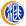500)this.width=500 align=center hspace=10 vspace=10 rel=nofollow/>

500)this.width=500 align=center hspace=10 vspace=10 rel=nofollow/500)this.width=500 align=center hspace=10 vspace=10 rel=nofollow/>

500)this.width=500 align=center hspace=10 vspace=10 rel=nofollow/500)this.width=500 align=center hspace=10 vspace=10 rel=nofollow/>

500)this.width=500 align=center hspace=10 vspace=10 rel=nofollow/500)this.width=500 align=center hspace=10 vspace=10 rel=nofollow/>

500)this.width=500 align=center hspace=10 vspace=10 rel=nofollow/

【以上观点属个人建议，仅供参考，据此交易风险自担。建议投资者以实际盘面走势具体分析应对。】【消息面】 时间消息及事件 05:00 美国至3月16日美联储利率决定 05:15 新西兰至3月16日联储利率决定 06:30 美联储主席鲍威尔召开线个大中城市住宅…